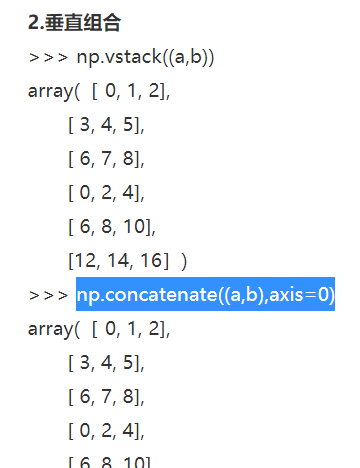• python传递参数方式小结

千次阅读 2021-01-14 15:55:01
本文实例总结了python传递参数方式。分享给大家供大家参考。具体分析如下：当形参如*arg时表示传入数组，当形参如**args时表示传入字典。输出：python中定义一个函数，可以通过正常的只传入值或key-value的方法调用...

本文实例总结了python传递参数方式。分享给大家供大家参考。具体分析如下：

当形参如*arg时表示传入数组，当形参如**args时表示传入字典。

输出：

python中定义一个函数，可以通过正常的只传入值或key-value的方法调用。但是如果第一个时参数传入的是key-value的方法，那么后面的必须都是key-value方法，如果第一个不是，那么后面的可以根据情况再传入值就可以了。

例子如下：

Python新手入门，在python中函式定义主要有四种方式：

① F(arg1,arg2,...)，最常见的定义方式，一个函式可以定义任何个参数，每个参数间用逗号分割，用这种参数在调用的时候必须在函式名后面的小括号中提供个数相等的值(实参)，并且顺序必须相同，形参与实参一一对应

调用a函式，a(1,2)则x=1,y=2，如果a(1)或者a(1,2,3)则会出错

② F(arg1,arg2=value2,...agrN=valueN)，则将为函式提供默认值。

调用该函式，a(1,2)则x=1,y=2，如果a(1)不会导致错误，此时x=1,y=3,y值将使用默认值，a(y=4,x=2)同理

可变参数：

③ F(*arg1),以一个*加形参的方式来表示函式的实参个数不确定，参数个数>=0，采用这样的方式定义函式，在函式内部将以实参名的方式构建一个元组(tuple)

遍历该元组(计算总和)的一种方式，此时r定义为一元组：

④ F(**arg)形参名前加2个**表示在函式内部将被存放在以形参名为标识符的dictionary，这时调用将使用arg1=value1，arg2=value2...

可通过以下方式来获取预期键值对，如果形参是未定义'y'的键，将返回None

Python参数调用过程按照以上四种方法优先级依次降低。

①方式解析，然后是②中的arg=value方式，再分别按照③>④优先级传参

这是自己第一份通过Blog整理的学习笔记，希望对自己，对浏览至此的各位朋友有所帮助，以上函式命名不符合规范，仅用于简单标识说明，使用python 2.6.2

希望本文所述对大家的Python程序设计有所帮助。

展开全文• 我们知道numpy.ndarray.reshape()是用来改变numpy数组的形状的，但是它的参数会有一些特殊的用法，这里我们进一步说明一下。代码如下：import numpy as npclass Debug:def __init__(self):self.array1 = np.ones(6)...

我们知道numpy.ndarray.reshape()是用来改变numpy数组的形状的，但是它的参数会有一些特殊的用法，这里我们进一步说明一下。代码如下：

import numpy as np

class Debug:

def __init__(self):

self.array1 = np.ones(6)

def mainProgram(self):

print("The value of array1 is: ")

print(self.array1)

print("The array2 is: ")

array2 = self.array1.reshape(2, 3)

print(array2)

if __name__ == '__main__':

main = Debug()

main.mainProgram()

"""

The value of array1 is:

[1. 1. 1. 1. 1. 1.]

The array2 is:

[[1. 1. 1.]

[1. 1. 1.]]

"""

这里我们看到我们将一个长度为6的一维数组变成了一个尺寸为(2, 3)的二维数组，这里的2代表2行，对应y轴，3代表3列，对应x轴。

然而有时候我们会在reshape中使用到-1这个参数，当使用这个参数时，会将数组重新塑形变得十分简单。代码如下：

class Debug:

def __init__(self):

self.array1 = np.ones(6)

def mainProgram(self):

print("The value of array1 is: ")

print(self.array1)

print("The array2 is: ")

array2 = self.array1.reshape(-1, 3)

print(array2)

if __name__ == '__main__':

main = Debug()

main.mainProgram()

"""

The value of array1 is:

[1. 1. 1. 1. 1. 1.]

The array2 is:

[[1. 1. 1.]

[1. 1. 1.]]

"""

我们可以看到当我们将reshape的第一个参数变为-1时，我们仍旧获得了一个尺寸为(2, 3)的数组，其实在这里，-1代表的意思为6 / 3 =2，其中6是被塑形一维数组的长度，3是我们指定的二维数组一个方向的维度。这样的好处就是当数据量比较大时，我们在二维数组重新塑形时只需要指定一个维度上的尺寸，另一个维度上的尺寸python会自动为我们计算。

到此这篇关于详解numpy.ndarray.reshape()函数的参数问题的文章就介绍到这了,更多相关numpy.ndarray.reshape()函数参数内容请搜索我们以前的文章或继续浏览下面的相关文章希望大家以后多多支持我们！

本文标题: 详解numpy.ndarray.reshape()函数的参数问题

本文地址: http://www.cppcns.com/jiaoben/python/353365.html

展开全文• ndarray基础操作

万次阅读 2019-02-07 22:45:01
ndarray是具有相同类型和大小的项目的（通常是固定大小的）多维容器。数组中的维和项的数量由其shape（形状）定义，该形状是指定每个维的大小的N个正整数的 元组 数组中的项类型由单独的数据类型对象（dtype）指定，...

N维数组

ndarray是具有相同类型和大小的项目的（通常是固定大小的）多维容器。数组中的维和项的数量由其shape（形状）定义，该形状是指定每个维的大小的N个正整数的 元组 数组中的项类型由单独的数据类型对象（dtype）指定，其中一个对象与每个ndarray关联。

与Python中的其他容器对象一样，可以通过对数组进行索引或切片(例如，使用整数n)，以及通过 ndarray 的方法和属性来访问和修改 ndarray 的内容。

不同的ndarrays可以共享相同的数据，因此在一个ndarray中所做的更改可能在另一个中可见。 也就是说，ndarray可以是另一个ndarray的“视图”，它所指的数据由“base”ndarray处理。 ndarrays也可以是Pythonchace所拥有的内存视图或实现buffer或数组接口的对象

数组属性

数组属性反映数组本身固有的信息。通常，通过数组的属性访问它，你可以获取并设置数组的内部属性，而无需创建新的数组。公开的属性是数组的核心部分，只有其中一些属性可以在不创建新数组的情况下进行有意义的重置。每个属性的信息如下。

内存相关的属性

以下属性包含有关数组内存的信息：

• ndarray.flags 有关数组内存分布的信息。
• ndarray.shape 数组维度的元组。
• ndarray.strides 遍历数组时要在每个维度中执行的字节元组。
• ndarray.ndim 数组维数。
• ndarray.data 指向数组数据开始的Python缓冲区对象。
• ndarray.size 数组中的元素数。
• ndarray.itemsize 一个数组元素的长度(以字节为单位)。
• ndarray.nbytes 数组元素消耗的总字节。
• ndarray.base 如果内存来自其他对象，则为基本对象。

数据类型

ndarray.dtype数组元素的数据类型。

其他属性

• ndarray.Tself.transpose()相同，只是如果 self.ndim <2 则返回自己。
• ndarray.real 数组的真实部分。
• ndarray.imag 数组的虚部。
• ndarray.flat 数组上的一维迭代器。
• ndarray.ctypes 一个简化数组与ctypes模块交互的对象。

数组方法

ndarray 对象有许多方法以某种方式对数组进行操作或与数组一起操作，通常返回数组结果。 下面简要说明这些方法。（每个方法的文档都有更完整的描述。）

对于以下方法，numpy中还有相应的函数：all，any，argmax，argmin，argpartition，argsort，choose，clip，compress，copy，cumprod，cumsum，diagonal，imag，max，mean，min，nonzero，partition， prod，ptp，put，ravel，real，repeat，reshape，round，searchsorted，sort，squeeze，std，sum，swapaxes，take，trace，transpose，var

数组转换

• ndarray.item(*args) 将数组元素复制到标准Python标量并返回它。
• ndarray.tolist() 将数组作为（可能是嵌套的）列表返回。
• ndarray.itemset(*args) 将标量插入数组（如果可能，将标量转换为数组的dtype）
• ndarray.tostring([order]) 构造包含数组中原始数据字节的Python字节。
• ndarray.tobytes([order]) 构造包含数组中原始数据字节的Python字节。
• ndarray.tofile(fid[, sep, format]) 将数组作为文本或二进制写入文件（默认）。
• ndarray.dump(file) 将数组的pickle转储到指定的文件。
• ndarray.dumps() 以字符串形式返回数组的pickle。
• ndarray.astype(dtype[, order, casting, …]) 数组的副本，强制转换为指定的类型。
• ndarray.byteswap([inplace]) 交换数组元素的字节
• ndarray.copy([order]) 返回数组的副本。
• ndarray.view([dtype, type]) 具有相同数据的数组的新视图。
• ndarray.getfield(dtype[, offset]) 返回给定数组的字段作为特定类型。
• ndarray.setflags([write, align, uic]) 分别设置数组标志WRITEABLE，ALIGNED，（WRITEBACKIFCOPY和UPDATEIFCOPY）。
• ndarray.fill(value) 使用标量值填充数组。

项目选择和操作

对于采用axis关键字的数组方法，默认为None。 如果axis为None，则将数组视为1维数组。轴的任何其他值表示操作应该沿着的维度。

• ndarray.take(indices[, axis, out, mode]) 返回由给定索引处的a元素组成的数组。
• ndarray.put(indices, values[, mode]) 为索引中的所有n设置 a.flat[n] = values[n]。
• ndarray.repeat(repeats[, axis]) 重复数组的元素。
• ndarray.choose(choices[, out, mode]) 使用索引数组从一组选项中构造新数组。
• ndarray.sort([axis, kind, order]) 就地对数组进行排序。
• ndarray.argsort([axis, kind, order]) 返回将对此数组进行排序的索引。
• ndarray.partition(kth[, axis, kind, order]) 重新排列数组中的元素，使得第k个位置的元素值处于排序数组中的位置。
• ndarray.argpartition(kth[, axis, kind, order]) 重新排列数组中的元素，使得第k个位置的元素值处于排序数组中的位置。
• ndarray.searchsorted(v[, side, sorter]) 查找应在其中插入v的元素以维护顺序的索引。
• ndarray.nonzero() 返回非零元素的索引。
• ndarray.compress(condition[, axis, out]) 沿给定轴返回此数组的选定切片。
• ndarray.diagonal([offset, axis1, axis2]) 返回指定的对角线。

计算

在下面这种情况下，其中许多方法都采用名为axis的参数。

• 如果axis为None（默认值），则将数组视为1-D数组，并对整个数组执行操作。 如果self是0维数组或数组标量，则此行为也是默认行为。 （数组标量是类型/类float32，float64等的实例，而0维数组是包含恰好一个数组标量的ndarray实例。）
• 如果axis是整数，则操作在给定轴上完成（对于可沿给定轴创建的每个1维的子阵列）。若数组是二维的，则axis=0表示沿列进行运算，axis=1表示沿行进行运算。

对于多种方法，还可以提供可选的out参数，并将结果放入给定的输出数组中。 out参数必须是 ndarray 并且具有相同数量的元素。 它可以具有不同的数据类型，在这种情况下将执行转换。

• ndarray.argmax([axis, out]) 返回给定轴的最大值索引。
• ndarray.min([axis, out, keepdims]) 沿给定轴返回最小值。
• ndarray.argmin([axis, out]) 沿a的给定轴返回最小值的索引。
• ndarray.ptp([axis, out]) 沿给定轴的峰峰值（最大值 - 最小值）。
• ndarray.clip([min, max, out]) 返回其值限制为 [min, max] 的数组。
• ndarray.conj() 复合共轭所有元素。
• ndarray.round([decimals, out]) 返回a，每个元素四舍五入到给定的小数位数。
• ndarray.trace([offset, axis1, axis2, dtype, out]) 返回数组对角线的总和。
• ndarray.sum([axis, dtype, out, keepdims]) 返回给定轴上的数组元素的总和。
• ndarray.cumsum([axis, dtype, out]) 返回给定轴上元素的累积和。
• ndarray.mean([axis, dtype, out, keepdims]) 返回给定轴上数组元素的平均值。
• ndarray.var([axis, dtype, out, ddof, keepdims]) 返回给定轴的数组元素的方差。
• ndarray.std([axis, dtype, out, ddof, keepdims]) 返回给定轴的数组元素的标准偏差。
• ndarray.prod([axis, dtype, out, keepdims]) 返回给定轴上的数组元素的乘积
• ndarray.cumprod([axis, dtype, out]) 返回沿给定轴的元素的累积乘积。
• ndarray.all([axis, out, keepdims]) 如果所有元素都计算为True，则返回True。
• ndarray.any([axis, out, keepdims]) 如果求值的任何元素为True，则返回True。

特殊方法

标准库函数：

• ndarray.copy() 如果在数组上调用copy.copy，则使用此方法。
• ndarray.deepcopy(memo, /) 如果在数组上调用copy.deepcopy，则使用此方法。
• ndarray.reduce() 用于腌制（译者注：很形象）。
• ndarray.setstate(state, /) 用于反腌制。

基本的定制：

• ndarray.new($type, args, *kwargs) 创建并返回一个新对象。 • ndarray.array(|dtype) 如果没有给出dtype，则返回对self的新引用;如果dtype与数组的当前dtype不同，则返回提供的数据类型的新数组。 • ndarray.array_wrap(obj) 容器的定制: (参见索引） • ndarray.len($self, /) 返回 len(self).
• ndarray.getitem($self, key, /) 返回 self[key]. • ndarray.setitem($self, key, value, /) 给 self[key] 设置一个值。
• ndarray.contains($self, key, /) 返回 自身的关键索引。 转换;操作complex，int，long，float，oct和hex。它们位于数组中，其中包含一个元素并返回相应的标量。 • ndarray.int(self) • ndarray.long • ndarray.float(self) • ndarray.oct • ndarray.hex 字符串表示： • ndarray.str($self, /) 返回 str(self).
• ndarray.repr(\$self, /) 返回 repr(self).

以上内容摘自https://www.numpy.org.cn/reference/array_objects/n_dimensional_array.html

展开全文Numpy ndarray
• 仅作为记录，大佬请跳过。 参考：传送门 用np.concatenate((a,b),axis=0)

仅作为记录，大佬请跳过。

参考：传送门

np.concatenate((a,b),axis=0)展开全文• 我们在描述一个多维数组的时候，经常会用到两个参数：维度（dimension）和形状（shape），以二维数组为例，我们可以用矩阵来形象地联想这两个参数，对于矩阵，显然我们最常见的矩阵是二维的，所以他的dim参数就是2，...
•numpy ndarray numpy总结
• list和numpy.ndarray切片赋值之不同 list lst = [1, 2, 3] temp1 = lst[1:] temp1 = 10 print(lst) print('-' * 20) temp2 = lst[1:] # temp[:] = 0 # 会出现错误 temp2[:] = [0, 0] print(temp[:]) print(lst) ...numpy python list 切片 赋值
• NumPy ndarray

2021-01-18 14:27:31
NumPy ndarray import numpy as np # NumPy N-dimensional array(N-维数组)： # NumPy提供一个N-维数组类型，ndarray 描述一个相同类型的多数据项的集合；多个数据项可以通过索引访问 # 所有ndarrays是同质的：每...
• Python3NumPy——ndarray对象1.前沿推荐导入语法：import numpy as npNumPy中使用ndarray对象表示数组，ndarray是NumPy库的核心对象2.创建ndarray对象函数array()传递Python序列创建数组import numpy as np #导入...
• ndarray.view([dtype][, type]) 官方文档 不改变数据域，设置dtype和type，返回更改后的数组 dtype: data-type or ndarray sub-class, optional 数据的类型, e.g., float32 or int16. 如果被传参数则保持不变 type：...python
• 操作Ndarray对象 一、创建Ndarray数组对象 说明 numpy默认ndarray的所有元素的类型是相同的，这一点与Python中的list不同 如果传进来的列表中包含不同的类型，则统一为同一类型，优先级：str>...参数 说明python r语言 开发语言
• 一、ndarray.transpose的官方文档： ndarray.transpose(*axes) Returns a view of the array with axes transposed. //返回对array进行维度转置后的结果 For a 1-D array this has no effect, as a transposed ...
• MXNet中的NDArray

2021-04-11 08:12:51
想要跑代码可以参考这里搭建环境，...  首先从MXNet导入ndarray模块，nd是ndarray的缩写形式，两种导入方式均可，但要注意之后使用的时候前缀要对应导入的形式。 from mxnet import ndarray #之后要使用ndarray机器学习 python mxnet
• like( ndarray ) 生成一个形状与参数相同的全一ndarray np.zeros( N) 生成一个N长度的一维全零ndarray np.zeros( N, dtype) 生成一个N长度类型位dtype的一维全零ndarray np.zeros_like(ndarray) 类似np.ones_like( ...
• NumPy ndarray数组的创建

千次阅读 2020-12-24 09:06:59
从已有数据中创建数组 直接对 Python 的基础数据类型（如列表、元组等）进行转换来生成 ndarray： 1) 将列表转换成 ndarray： import numpy as np ls1 = [10, 42, 0, -17, 30] nd1 =np.array(ls1) print(nd1) print...
• ndarray 依赖 Cargo.toml 文件加入： [dependencies] ndarray = "0.15.3" ndarray 具体函数可参考 https://blog.csdn.net/wsp_1138886114/article/details/108635600 一、向量Vec 与 Array互转 extern crate ...
• Python高阶——Numpy创建ndarrayPython高阶——Numpy创建ndarray如何导入NumpyPython 列表和 NumPy 阵列有什么区别用np.array() 创建一个 ndarray对象创建数组1、np.empty()创建指定形状、数据类型，且没有初始化的...开发语言 python
• 返回的对象除其他外，还具有数据，形状和步幅属性(请参阅下面的注释)，它们本身返回ctypes对象，这些对象可用作共享库的参数参数：None：返回值：c： ： Python对象拥有属性数据，形状，步幅等注意：以下是在...
• Numpy库（一）：创建ndarray 一、Numpy库简介 二、ndarray对象 （1）创建数组 （2）数组常用属性 （3）数组的数据类型 （4）内置特殊数组创建形式 1.零一数组、对角数组 2.序列数组 3.随机数组 一、Numpy库简介 ...
• mxnet NDArray

2021-01-06 17:15:20
MXNet的NDArray与Numpy中的ndarray极为相似，NDAarray为MXNet中的各种数学计算提供了核心的数据结构，NDArray表示一个多维的、固定大小的数组，并且支持异构计算。那为什么不直接使用Numpy呢？MXNet的NDArray提供...
• Numpy库的核心是ndarray，实际上就是N维数组（N-dimensional array），关于这个数据对象的详细介绍，参考官方文档最为合适。有一点要注意的是，ndarray的内置方法只有30多个，常用的如求平均值可以写a.mean()，但是...python numpy ndarray axis axes
• ndarray中保留小数的方法

千次阅读 多人点赞 2021-05-19 09:42:55
ndarray中保留小数的方法np.set_printoptions()np.around() 前言： 作者：神的孩子在跳舞 本人是刚开始学机器学习的小白，以下都是我的学习笔记，有一些是我自己理解的话，所以可能有不对的地方或者有些话只适合我...python 机器学习 numpy
• 参数：new_shape： ： tuple of ints, 或 n ints调整大小后的数组的形状。refcheck： ： bool, 可选参数如果为False，则不会检查引用计数。默认值为True。返回值：None：异常：ValueError：如果a不拥有自己的数据或...
• 带有标量的计算：把计算参数传递给数组的每一个元素。 同尺寸数组之间的比较：产生一个布尔值数组。 不同尺寸数组之间的操作：使用广播特性，这是进阶部分，后面可能会补充。 【例1】同尺寸数组之间的计算示例 In ...python 矩阵 开发语言 numpy
• #ndarray的运算 #ndarray 多维_同类_数据容器 #numpy的ndarray的相加相乘，与python的元组、列表不一样，而与matlab保持一致。 data = np.random.randn(2,3) print(data) print(data * 10) print(data + data) print...python
• ndarray对象是用于存放同类型元素的多维数组，是numpy中的基本对象之一，另一个是func对象。本文主要内容是：1 、简单介绍ndarray对象；2、ndarray对象的常用属性；3、如何创建ndarray对象；4、ndarray元素访问。 它...
• NumPy ndarray数组元素的获取

千次阅读 2020-12-24 06:02:31
生成 ndarray 数组后，如何读取我们所需要的数据呢？在 NumPy 中，既可以获取 ndarray 数组的单个元素，也可以获取一组元素(也即切片)，这与 Python 中的列表(list)和元组(tuple)非常类似。请看下面的代码：import ...numpy 数组抽取
• 对秩为1的两个ndarray进行加减乘除import numpy as np# 创建2个秩为1的ndarrayx = np.array([1,2,3,4])y = np.array([5.1,6.1,7.1,8.1])# 打印xprint('x = ', x)# 打印yprint('y = ', y)# 通过两种不同的方式，对x和...
• 子类化的ndarray相对简单，但是与其它Python对象相比，它却更复杂一点。 ndarrays和对象创建 ndarray的子类化很复杂，因为ndarray类的新实例可以通过三种不同的方式产生。 1.显示的构造函数调用在MySubClass（params...python numpy 编程语言 程序人生 人工智能
• _like() 的函数创建与参数数组的形状及类型相同的数组。 frombuffer()、fromstring()、fromfile() 等函数可以从字节序列或文件创建数组 4、np.arange | 方法 | 描 述 | arange([start,] stop[, step,][, ......